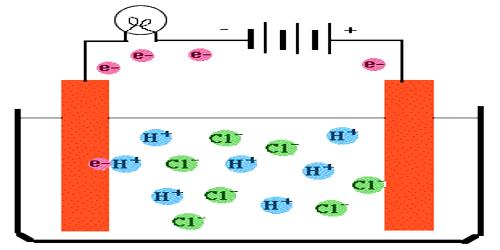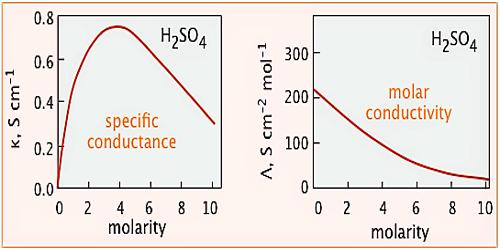Chemistry

# Conductance of Electrolytic SolutionsThe Conductance of Electrolytic Solutions

The power of conducting electricity by any conductor is described in terms of its conductivity or conductance. Conductance is reciprocal of resistance. If the electrical resistance of a conductor is measured the conductance may be calculated as

Conductance = 1 / Resistance

The resistance of an electrical conductor may be measured by using Ohm’s law written as:

R = E/I

where R is the resistance measured in ohm(Ω), I is the current in ampere and E is the potential difference (volts) between the two ends. This law can be applied in case of solutions except at very high voltages or with very high frequency alternating current.

In order to be able to compare the resistances of different substances the term specific resistance has been introduced. The resistance, R, of a conductor is directly proportional to its length, l, and inversely proportional to its area of cross-section, a, i.e.,

R ∞ l/a

Or, R = ρ x (l/a) … …. … (1)

where ρ (rho) is a constant for the conducting material and is called its specific resistance or resistivity. When l = 1 cm and a = 1 sq cm, R = ρ. The specific resistance is then defined as follows:

The specific resistance of a substance is the resistance in ohms between the opposite faces of a cube of the material having an edge one centimeter long.The unit of specific resistance can he found from equation (1)

ρ = (Rxa)/l = (ohm x cm2) / cm = ohm – cm    (Ω – cm)

The reciprocal of resistance is called conductance, Λ (lambda)

Λ = 1/R = 1/ohm = ohm-1-1)

The reciprocal of specific resistance is known as the specific conductance, κ (kappa).

By definition, specific resistance (κ) = 1/ρ = (1/R) x (l/a)

The unit of specific conductance can be derived as follows:

specific conductance, κ =  1/ρ = (1/R) x (l/a) = 1/ohm x cm/cm2 = ohm-1 cm-1

The specific conductance of a solution is dependent on its concentration. In order to compare the conductance of different electrolytic solutions, molar conductance (Λm) is used. The molar conductance is defined as-

“The conductance of all the ions produced when 1 mole of an electrolyte is dissociated into its ions in a volume V mL”.

This is obtained by multiplying specific conductance (κ) by the volume V in mL that contains 1 mole of the electrolyte. In other words,

Λm = κV

Here, V is the volume of the solution in mL containing 1 mole of the electrolyte.